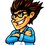All answers are integer values from 0 to 999Note by Mark Mottian
6 years, 2 months ago

This discussion board is a place to discuss our Daily Challenges and the math and science related to those challenges. Explanations are more than just a solution — they should explain the steps and thinking strategies that you used to obtain the solution. Comments should further the discussion of math and science.

When posting on Brilliant:

• Use the emojis to react to an explanation, whether you're congratulating a job well done , or just really confused .
• Ask specific questions about the challenge or the steps in somebody's explanation. Well-posed questions can add a lot to the discussion, but posting "I don't understand!" doesn't help anyone.
• Try to contribute something new to the discussion, whether it is an extension, generalization or other idea related to the challenge.

MarkdownAppears as
*italics* or _italics_ italics
**bold** or __bold__ bold
- bulleted- list
• bulleted
• list
1. numbered2. list
1. numbered
2. list
Note: you must add a full line of space before and after lists for them to show up correctly
paragraph 1paragraph 2

paragraph 1

paragraph 2

[example link](https://brilliant.org)example link
> This is a quote
This is a quote
    # I indented these lines
# 4 spaces, and now they show
# up as a code block.

print "hello world"
# I indented these lines
# 4 spaces, and now they show
# up as a code block.

print "hello world"
MathAppears as
Remember to wrap math in $$ ... $$ or $ ... $ to ensure proper formatting.
2 \times 3 $2 \times 3$
2^{34} $2^{34}$
a_{i-1} $a_{i-1}$
\frac{2}{3} $\frac{2}{3}$
\sqrt{2} $\sqrt{2}$
\sum_{i=1}^3 $\sum_{i=1}^3$
\sin \theta $\sin \theta$
\boxed{123} $\boxed{123}$

Sort by:

Question 20

How many different numbers can be written as the product of two or more of the numbers 3, 4, 4, 5, 5, 6, 7, 7, 7 ?

- 6 years, 2 months ago

A simple way to approach this question is to write the numbers out in a grid, and then multiply them out while ignoring terms in the main diagonal.

Staff - 6 years, 2 months ago

Hi Calvin. Thanks for the response. Won't the grid only work for determining the number of unique products if exactly TWO numbers are chosen? How can you determine the number of unique products if three or more numbers are chosen and multiplied?

- 6 years, 2 months ago

oh ooops, did not see "or more". That makes it much easier then.

Claim: Each product can be uniquely written as $3^a \times 4^b \times5 ^c \times 6 ^ d \times 7 ^ e$, where $0 \leq a \leq 1, 0 \leq b \leq 2, 0 \leq c \leq 2, 0 \leq d \leq 1, 0 \leq e \leq 3$. This happens to work for the set of numbers, and it should be rigorously proved. It would not work if we had 2 3's and 2 6's.

So, there are $2 \times 3 \times 3 \times 2 \times 4$ possible products. The number of products of 0 terms is 1. The number of products of 1 term is 5. So subtract $1 +5 = 6$ from the previous total.

Staff - 6 years, 2 months ago

Question 19

ABCDE is an irregular pentagon with DC = DE and the angles at E and C are right angles. Angle DAB is 70 degrees and angle DBA is 60 degrees. Also, EA + BC = AB. Determine the size of the angle EDC in degrees.

- 6 years, 2 months ago

Question 7

The nine digits 1, 2, 3, 4, 5, 6, 7, 8 and 9 are each used exactly once in writing three 3-digit numbers. In each of the three numbers, the middle digit is the largest. What is the smallest possible sum of these three numbers?

- 6 years, 2 months ago

The priority is getting the lowest hundred's digits, then the lowest tens digits and then the lowest ones digits.

So The numbers should be $1 \_ \_, 2 \_ \_, 3 \_ \_$. Next, we need to put the tens digits. We can't put a 4, because then we can't put anything in the ones place. But we can put a 5; putting a 4 in the ones place. Likewise, we can't place a 6, since both 5,4 are used, but we can put a 7. Like wise for 8,9. Putting them in place, we get $154,276,398$ with a sum of $\boxed{828}$

- 6 years, 2 months ago

- 6 years, 2 months ago

Question 16

Let f be a function satisfying:

$f(xy) = f(x)/y$

for all positive real numbers x and y. If $f(500) = 3$, what is the value of $f(100)$?

- 6 years, 2 months ago

Isn't this a one line solution?

$f(5*100) = f(100)/5 \implies f(100) = 5*f(500) = \boxed{15}$

- 6 years, 2 months ago

Hi Siddhartha. As usual, it's an absolute pleasure to discuss things with you. Yes, that's my exact solution!

- 6 years, 2 months ago